# The QUANTREG Procedure

### Leverage Point and Outlier Detection

The QUANTREG procedure uses robust multivariate location and scale estimates for leverage-point detection.

Mahalanobis distance is defined as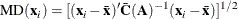where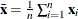and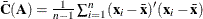are the empirical multivariate location and scale, respectively. Here,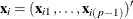does not include the intercept variable. The relationship between the Mahalanobis distance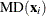and the matrix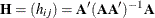is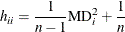Robust distance is defined as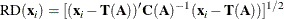where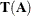and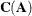are robust multivariate location and scale estimates that are computed according to the minimum covariance determinant (MCD) method of Rousseeuw and Van Driessen (1999).

These distances are used to detect leverage points. You can use the LEVERAGE and DIAGNOSTICS options in the MODEL statement to request leverage-point and outlier diagnostics, respectively. Two new variables, Leverage and Outlier, respectively, are created and saved in an output data set that is specified in the OUTPUT statement.

Let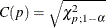be the cutoff value. The variable LEVERAGE is defined as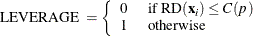You can specify a cutoff value in the LEVERAGE option in the MODEL statement.

Residuals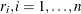, that are based on quantile regression estimates are used to detect vertical outliers. The variable OUTLIER is defined as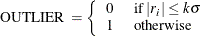You can specify the multiplier k of the cutoff value in the CUTOFF= option in the MODEL statement. You can specify the scale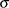in the SCALE= option in the MODEL statement. By default, k = 3 and the scaleis computed as the corrected median of the absolute residuals: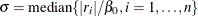where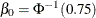is an adjustment constant for consistency when the normal distribution is used.

An ODS table called DIAGNOSTICS contains the Leverage and Outlier variables.